##### Circuit Analysis For DummiesIf you understand the basic building blocks of op amp circuits, you’re ready to tackle complex processing actions with op amps. Using op amp circuits, you can analyze an instrumentation amplifier, solve mathematical equations, or create systems for signal processing, instrumentation, filtering, process control, or digital-to-analog/analog-to-digital conversion.

## Analyze an instrumentation amplifier

The instrumentation amplifier is a differential amplifier suited for measurement and test equipment. Here is the input stage of an instrumentation amplifier. Your goal is to find the voltage output vO proportional to the difference of the two inputs, v1 and v2. Getting the desired output requires some algebraic gymnastics, but you can handle it.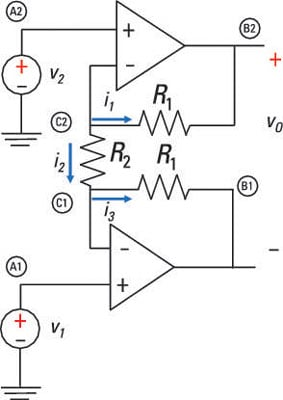At Node C2, you apply KCL (i1 + i2 = 0) and Ohm’s law (i = v/R) and wind up with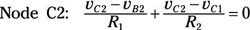At Node C1, the KCL equation (–i2 + i3 = 0) with Ohm’s law leads you to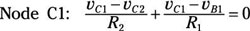The sample circuit shows the noninverting input connected to independent voltages v1 and v2. Use the op amp voltage constraint vP = vN to get the following: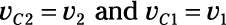Substitute v1 and v2 into KCL equations, which gives you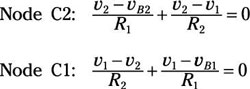Now solve for vB2 and vB1, because the output voltage vO depends on these two values: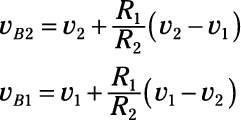The output voltage vO is the difference between the vB1 and vB2: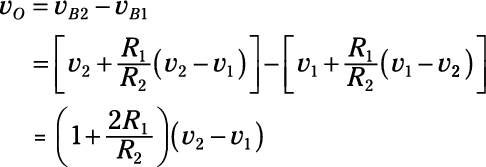Cool! Resistor R2 can be used to amplify the difference v2v1. After all, it’s easier to change the value of one resistor R2 than of two resistors R1.

## Implement mathematical equations electronically

As an example of how op amps can solve equations, consider a single output and three voltage input signals: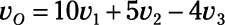You can rewrite the equation in many ways to determine which op amp circuits you need to perform the math. Here’s one way: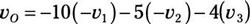The equation suggests that you have an inverting summer with three inputs: –v1, –v2, and v3. You need an inverting amplifier with a gain of –1 for v1 and v2. Input v1 has a summing gain of –10, input v2 has a summing gain of –5, and input v3 has a summing gain of –4.

You can see one of many possible op amp circuits in the top diagram of this sample circuit. The dashed boxes indicate the two inverting amplifiers and the inverting summer.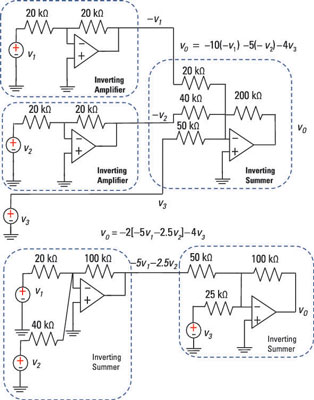The outputs of the two inverting amplifiers are – v1 and – v2, and they’re inputs to the inverting summer. The third input to the summer is v3. Adding up the three inputs with required gains entails an inverting summer, which you see in the sample circuit.

For input v1, the ratio of the inverting summer’s feedback resistor of 200 kΩ to its input resistor of 20 kΩ provides a gain of –10. Similarly, for input v2, the ratio of the feedback resistor of 200 kΩ to its input resistor of 40 kΩ gives you a gain of –5.

Finally, for input v3, the ratio of the feedback resistor of 200 kΩ to its input resistor of 50 kΩ provides a gain of –4. You can use other possible resistor values as long as the ratio of resistors provides the correct gains for each input.

Reducing the number of op amps during the design process helps lower costs. And with some creativity, you can reduce the number of op amps in the circuit by rewriting the math equation of the input-output relationship: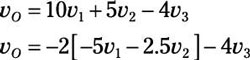This suggests you need two op amps. One input is a combination of inputs v1 and v2 formed by an inverting summer. When you take the output of the first summer and feed it and another input to a second inverting summer, the result is proportional to v3 with gain –4. The bottom diagram of the sample circuit shows one way to implement this equation.

For v1, the ratio of the feedback resistor of 100 kΩ to the input resistor of 20 kΩ produces a gain of –5. For v2, the input resistor of 40 kΩ gives you a gain of –2.5. The output of the first summer is then multiplied by –2 because of the ratio of the second inverting summer’s feedback resistor of 100 kΩ to the input resistor of 50 kΩ.

The input v3 to the second summer is multiplied by –4 because of the ratio between the 100-kΩ feedback resistor to the 25-kΩ resistor.

## Create systems with op amps

Op amp circuits are basic building blocks for many applications in signal processing, instrumentation, process control, filtering, digital-to-analog conversion, and analog-to-digital conversion.

For example, you can do a digital-to-analog conversion (DAC) using the inverting summer. The primary purpose of this common device is to convert a digital signal consisting of binary 1s and 0s (perhaps coming from your personal computer) to an analog and continuous signal (to run your DC motor in your remote-control toy). The device has extensive applications in robotics, high-definition televisions, and cellphones.

This example is simplified by focusing on 3-bit devices (even though most applications use 8- to 24-bit DACs). DACs have one output voltage vo with a number of digital inputs (b0, b1, b2), along with a reference voltage VREF. Here you see a block diagram of a 3-bit input.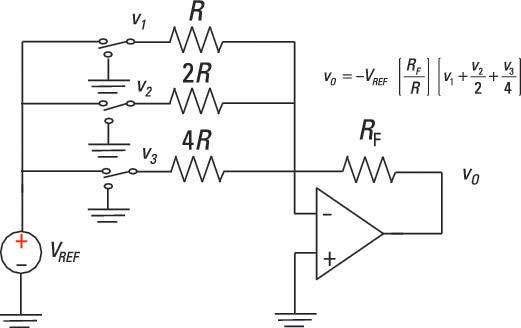The following equation gives you the relationship between the digital input and analog output: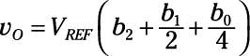Bit b2 is the most significant bit (MSB) because it’s weighted with the largest weight in the sum; bit b0 is the least significant bit (LSB) because it has the smallest weight.

To implement a DAC, you can use an inverting summer, as shown in the figure. Also shown are the digital inputs that can have only one of two voltage values: A digital 1 is equal to VREF, and a digital 0 is equal to 0 volts.

The inputs v1, v2, and v3 to the summer are weighted appropriately to give you the voltage output vO based on the three inputs. Input v1 has the most weight, and input v3 has the least.